telescopeѲptics.net          ▪▪▪▪                                             CONTENTS

# 1.1. POINT SPREAD FUNCTION (PSF)

As the previous page implies, diffraction of light in a perfect aperture is merely the limiting case of light interference from a circular area of wave emitters (which can be assumed as filling the telescope aperture), with wavefront aberrations and pupil obstructions approaching zero. Presence of significant wavefront aberrations, or pupil obstruction, alters wave interference and thus the intensity distribution and visual appearance of the diffraction pattern as well. In general, the effect is worsening of the image quality.

And, as we've seen, even in the best possible scenario, i.e. in the absence of aberrations and pupil obstruction, the image of a point object is not a point. Rather, it is a pattern of energy distribution consisting of the bright central disc surrounded by rapidly fainting concentric rings. This is the diffraction image of a point source in a clear aberration-free aperture. Its mathematical description is Point Spread Function (PSF), which expresses the normalized intensity distribution of the point-source image (it should be noted that this diffraction PSF is different from the geometrical PSF, which is based on the distribution of rays in point image). The actual energy distribution of the point-object image is given by a product of the PSF and the total power in the pupil.

Before going to the properties of the diffraction PSF, briefly about the definition of a point-object.

In general, any object whose Gaussian (geometric) image is smaller than the central maxima of the diffraction pattern - Airy disc - is considered to be a point-source. Be it physically as small as an atom, or as enormously large as a star. In other words, such object needs to feet into a cone outlined by drawing lines from the top and bottom of the Airy disc in the focal plane through the center of a pupil placed at the distance equal to the focal length in front of it. From similar triangles, point-source needs to be smaller than sA/f, s being the object distance, A the Airy disc diameter and f the focal length.

More accurately, according to the optical theory, a true point-source has to be less than 1/4 of the Airy disc in diameter; larger source will appreciably modify the "perfect" PSF by enlarging the central maxima, and altering (suppressing) diffraction rings (at the image size of ~0.25 Airy disc diameter, the FWHM is enlarged ~2%, at twice that size it is about 8% larger, and with the source equaling the Airy disc in diameter the FWHM is nearly doubled, and the ring structure greatly suppressed).

This is a good example of why we do need to know, very specifically, where does the light energy go. And for that we need diffraction calculation, which integrates wave contribution from every point (actually, very small area) in the pupil to any point in the image space. This is, generally, described by some form of diffraction integral. By incorporating in such integral the effect of aberrations or pupil obstructions on the wave contribution to image points, such integral can also describe the effect of aberrations and obstructions on the energy distribution in the diffraction image. Those are the basics of physical optics. For now, we limit to the clear aberration-free aperture.

While the visual PSF simulation is informative to some extent, it is its exact mathematical description that enables us to determine performance limits set by a "perfect aperture". Conventionally accepted limit to the point-image resolution set by diffraction equals the full width at half-maximum (FWHM) of the diffraction PSF, given angularly by /D in radians (with 1 radian equaling 180/π degrees, the FWHM is 648,000λ/Dπ in arc seconds, or 180λ/Dπ in degrees), D being the aperture diameter, and λ the wavelength of light. For contrast and resolution of extended images, diffraction PSF is the basis for obtaining modulation (MTF) and contrast (CTF) transfer functions.

Follow detailed description of the diffraction PSF and its properties. Inset E shows aberration-free PSF in-focus and longitudinal intensity distribution for clear circular aperture. Inset F shows the encircled energy, inset G the amplitude (coherent) spread function, its relation with the PSF and differences in image formation for incoherent and coherent light, inset H the effect of of spectral bandwidth on PSF, inset I illustrates the difference between the nominal and apparent (to eye) PSF intensity distribution, and inset J shows the effect of pupil (aperture) form on the PSF.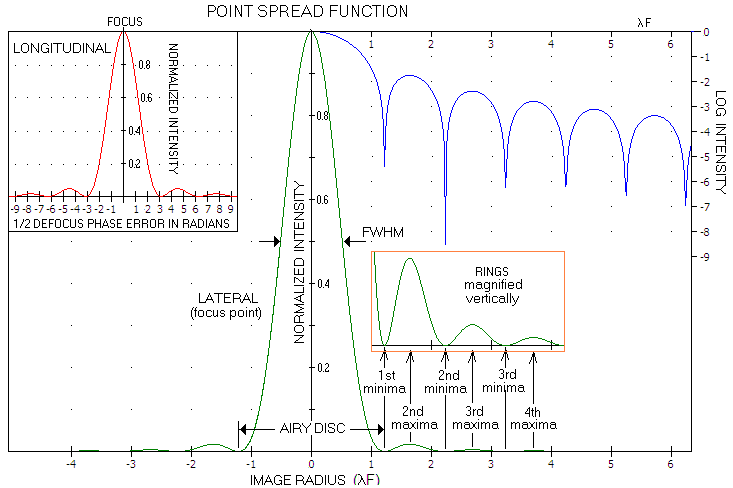INSET E: PSF of a clear aberration-free aperture. Since the ring intensity is much lower than that of the central maxima, PSF is often graphically presented in logarithmic form, i.e. as log I(r) (blue). In the direction of light propagation, PSF is located at the point of longitudinal maxima, in the plane perpendicular to it. The longitudinal energy spread (red, top left) is very similar in form, given by a sinc function I(l)=(sinφ/φ)2, with φ - the variable on the horizontal scale - being one half of the nominal defocus phase error, the latter equaling the P-V wavefront error in units of the wavelength multiplied by 2π (radians; so, φ=π equals 1 wave P-V wavefront error). This normalized longitudinal intensity equals the Strehl, and reaches 1 - same as the peak PSF value - at the focus location. The exact relation describing longitudinal energy spread includes multiplying factor (1+δ)2, where δ is the defocus in units of focal length; for most any telescope this factor can be neglected in the usual range of defocus error (note that this symmetrical longitudinal distribution is valid for Fresnel number value - given as N=D/4λF - of ~100 and larger, a condition easily fulfilled with any telescope).

Intensity at any point of the pattern in the plane of focus for either coherent or near-monochromatic incoherent point-source, normalized to 1 for peak intensity at the center is given by: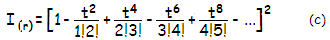with t=πr/2 (to simplify the relation), r being the point radius in the image plane, in units of λF (n!, the factorial, is a product of all integers smaller or equal to n; for example, 3!=3x2x1, and 5!=5x4x3x2x1). Note that the form of a term in the series can be generalized as tn/(0.5n)!(0.5n+1)!, with n=2, 4, 6, 8...

While straightforward, PSF calculation is cumbersome, involving large numbers in both, numerator and denominator for points farther from the center (up to the 3rd bright ring, 16 terms in addition to 1 are needed, up to the 2nd 12, up to the 1st 8, and for the central maxima four). Eq. (c) is derived from the general PSF relation for circular aperture,

I(r)=[2J1(πr)/πr]2, where

J
1(πr)=[1-(t2/1!2!)+(t4/2!3!)-(t6/3!4!)+...]t
is the first order Bessel function of the first kind of
πr, thus
2J
1(πr)/πr = 2[1-(t2/1!2!)+...]t/πr = [1-(t2/1!2!)+...].
The dependence on
λF in r indicates that the actual linear pattern size scales with both, wavelength and focal ratio. Its corresponding angular size is in λF/f=λ/D radians (f being the focal length and D the aperture diameter), thus changing in proportion to the wavelength, and in inverse proportion to aperture diameter.

Alternately, point radius r in the image plane can be expressed in terms of radians of the phase difference corresponding to it, as rR=(kDsinα)/2=rπ, where k=2π/λ is the wave propagation number (expressing the number of cycles in radians per mm),
D
is the aperture diameter and sin
α=rλF/f=rλ/D the angular point radius, with the consecutive PSF minimas  falling at 3.83, 5.14, 7.02... With the full phase being 2π radians, the appropriate values in units of phase are 0.61, 0.818, 1.117...

While Eq. (c) implies that the linear, or transverse size of diffraction pattern changes in proportion to the telescope's
F-number, angular size of the pattern changes in inverse proportion to the aperture diameter. As a function of the angular pattern size, equation for the normalized PSF intensity can be written as: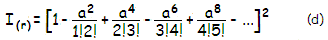with a=πDsinα/2, in units of λ, where D is the aperture diameter and α the angular point height in the image plane. Obviously, a is numerically identical to t, so for the first minima (Airy disc radius), the corresponding function value is 2a=πDsinα/λ=1.22π, with sinα=1.22λ/D (which is for very small angles identical to the angle in radians), changing in inverse proportion with the aperture diameter. Or, quite simply, α=rλF/f=rλ/D in radians. For D=100mm and λ=0.00055mm, angular radius of the first minima (r=1.22) is α=1.22∙0.00055/100=0.00000671 in radians or, multiplied by 206,265 (for 180/π degrees in 1 radian x 60 arc minutes in 1 degree x 60 arc seconds in 1 arc minute), 1.384 arc seconds.

INSET F: The diameter of the first PSF minima, given by 2.44λF linearly and 2.44λ/D angularly (in radians) - λ being the wavelength of light, and F the ratio of focal length vs. aperture D of the optical system - is called Airy disc diameter. With the normalized (to 1) encircled energy (EE) within pattern radius r in units of λF given by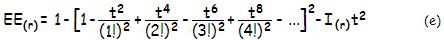(sum in the brackets being zero-order Bessel function, J0(πr), and t=πr/2, as before), it encircles 0.838 of the total energy contained in the diffraction pattern. Note that I(r)t2 = [J1(πr)]2, or the
1st-order Bessel function of
πr squared, implying the general relation form EE(r) = 1 - [J0(πr)]2 - [J1(πr)]2. With J(1) being practically zero at every minima for near-monochromatic light, as long as well defined pattern of intensity change exists, the encircled energy within any given minima radius reduces to 1-[J0(πr)]2, and the remaining energy outside that radius to [J0(πr)]2. As the diffraction plots to the right indicate, energy spread caused by diffraction extends far beyond the first few bright rings. According to Schroeder's EE approximation for larger r values, EE~1-(2/rπ2), about 2% of the total energy is still contained beyond the 10th dark ring. In amateur telescopes, however, this thinly stretched energy is below the threshold of detection in both, visual observing and astrophotography.

As the PSF and EE plots illustrate, the value of normalized encircled energy within 1st maxima is nearly inverse to the normalized intensity for r<0.8. Thus the encircled energy within 1st maxima, as a relative fraction of the total energy encircled within it, is well approximated as EE~1-I for r smaller than ~0.8. Taking Gaussian approximation of I, which is also good for r<0.8 gives EE~1-2-P, with P=3.77r2.

Following table gives numerical presentation of the intensity distribution up to 10th dark ring within diffraction pattern in near-monochromatic light by aberration-free unobstructed aperture, for linear radius r in units of λF, normalized intensity (I) and encircled energy (EE) (source: Optical Imaging and Aberrations 2, Mahajan).

 1st 2nd 3rd 4th 5th 6th 7th 8th 9th 10th max min max min max min max min max min max min max min max min max min max min r 0 1.22 1.63 2.23 2.68 3.24 3.70 4.24 4.71 5.24 5.72 6.24 6.72 7.25 7.73 8.25 8.73 9.25 9.73 10.25 I 1 0 0.0175 0 0.0042 0 0.0016 0 0.0008 0 0.0004 0 0.0003 0 0.0002 0 0.0001 0 0.0001 0 EE 0 0.838 0.867 0.910 0.922 0.938 0.944 0.952 0.957 0.961 0.964 0.968 0.970 0.972 0.974 0.975 0.977 0.978 0.979 0.98

Conventionally, the limit to diffraction resolution of two point-object images is set at ~λ/D, nearly identical to the
full width at half-maximum
(FWHM) of the PSF, 1.03λ/D radians in diameter.

Wave interference doesn't only occur radially; wavelets meeting before and after the focal point also interfere, extending the pattern of intensity longitudinally, generally decreasing as the interference takes place farther from the focal point. As a result, diffraction pattern is a 3-dimensional phenomenon. While relative intensities of the central disc vs. rings remain constant, as given above, visual appearance of diffraction pattern - the visibility of its segments, as well as their apparent size and relative apparent brightness - varies with the brightness of the point image.

Intensity distribution within the 1st PSF maxima (the bell-shaped central portion) is well approximated for r~0.8 and smaller by a Gaussian function of the form I~2-P, with P=(x2+y2)/r'2, where x and y are the point coordinates in horizontal plane (zero at the center), and r' the FWHM (full-width-at-half-maximum) radius, both in units of λF. Substituting for FWHM radius r'~1 and setting y to zero, with x effectively becoming r as defined with Eq. (c), gives the exponent for 2-D Gaussian central maxima approximation as P=3.77r2. For r>1 , Gaussian approximation asymptotically approaches horizontal axis, without any hint of the ring structure. Following table gives actual and approximated values for intensity distribution within central maxima of unobstructed aberration-free aperture:

 Radius r, in units of λF 0 0.2 0.4 0.515 (FWHM) 0.6 0.8 1 1.2 Actual normalized (to 1) intensity 1 0.9053 0.6645 0.5000 0.3806 0.1544 0.0328 0.0002 Gaussian approximation, I~2-P, P=3.77r2 1 0.9008 0.6584 0.5000 0.3904 0.1878 0.0733 0.0232

Note that this Gaussian is optimized for the best fit close to the center. Slightly different Gaussians can give better overall fit. The approximation used by Mahajan is I~exp[-(πr/2)2], with exp[x]=ex, e=2.718... being the natural logarithm base.

INSET G: The relative irradiance intensity distribution given by Eq. (c) applies to both, coherent and near-monochromatic incoherent light, since the light emitted by a point-source is also coherent if near-monochromatic. But there is important difference between the two in how the energy is generated, which is the reason for presenting spread function in its two distinct forms - the coherent spread function (CSF), given by the Bessel function in brackets of Eq. (c), and incoherent PSF, equal to the coherent spread function squared (calling PSF "incoherent" may be somewhat confusing, since it is essentially an image of coherent point-object; the rationale is in this energy distribution function being the building block of the image of an incoherent object).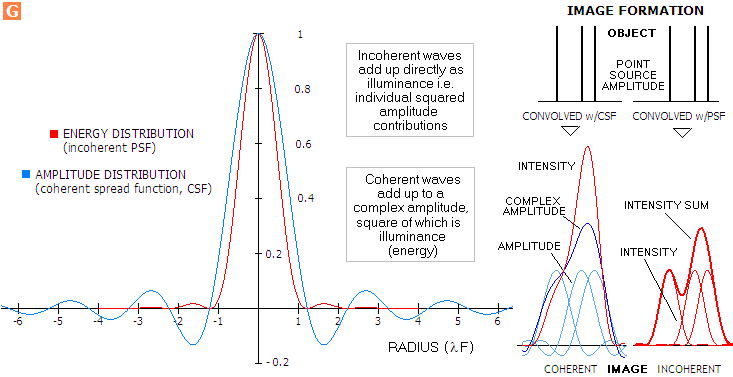With coherent light, due to constant phase relationship between individual waves, contributing amplitudes at each image point - the image being produced by overlapping CSF from every object point - first add up, and the intensity is this complex amplitude squared. With incoherent light, individual waves randomly vary in phase, preventing constructive amplitude interference; rather, individual wave intensities, as their individual amplitudes squared, add up at every image point directly as intensity contributions (Inset G). Hence, irradiance intensity of the diffraction image for given flux density is proportional to the square of pupil area for the former, and to the pupil area for the latter. This does not affect the relative intensity distribution of the point-object image (PSF), which are identical for either coherent or near-monochromatic incoherent light, but does result in a different intensity distribution for extended object image, as well as close point-object images. While such extended image in coherent light forms by summing up and squaring complex amplitude to obtain the energy (illuminance, equaling complex amplitude squared) at every point of the image, with incoherent light it is directly a sum of illuminance (energy) contributions at every image point, i.e. sum of point-images of object points (the reason being that coherent waves are correlated in phase, thus building up complex amplitude, while incoherent waves oscillate independently and only interfere at the energy level). As plots at right show, this difference in wave interference between the two will result in a different image characteristics of the same object. The three point-source object shown on top is partly resolved in incoherent light, but not in coherent (bottom).

INSET H: While, according to Van Cittert-Zernike Theorem, star light is coherent in amateur apertures, if near monochromatic, the actual (polychromatic) star light is closer to incoherent, and its PSF indicates somewhat different diffraction pattern, as shown below (light from extended astronomical objects is always incoherent). The central maxima is slightly expanded, and the rings are less pronounced, particularly beyond the first 2-3 bright rings. Encircled energy is somewhat lower due to enlarged central maxima, but nearly equilizes with that for monochromatic light farther out, due to more energy packed up in the dark ring areas.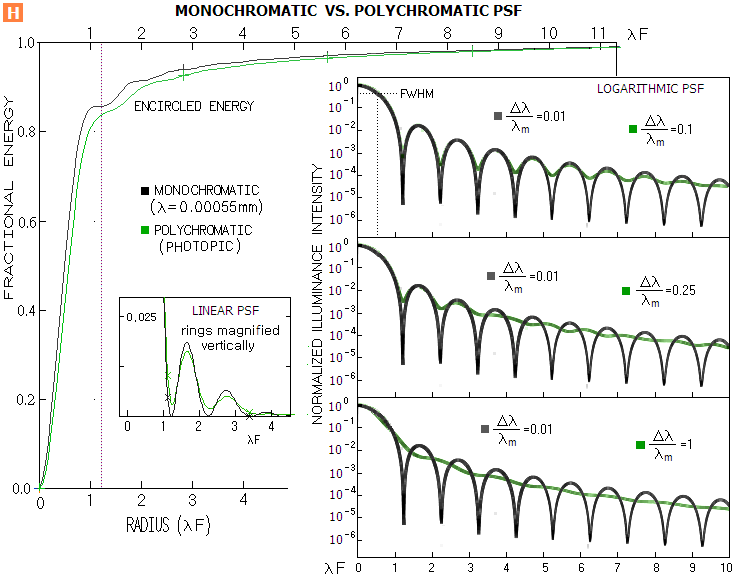Eq. (c)
places the first diffraction minima at r=1.22 (slightly rounded off, from 1.219668), second at  r=2.23, third at 3.24, and so on. Plots to the left ("Optical Imaging and Aberrations 2", Mahajan) show PSF intensity distribution on logarithmic (log
10) scale for aberration-free circular aperture, as a function of pattern radius r in units of λF, for object radiance constant over the range of radiation. The ring structure with the spectral range Δλ being 0.01 of the mean wavelength λm is still clearly pronounced, nearly identical to that for near-monochromatic light (Δλ~0.0001λm). It gradually vanishes as the range widens to Δλ~λm. Most astronomical objects emit in wide spectral ranges, but with the more  narrow peak intensity range. Thus, the typical pattern is intermediate between patterns for near-monochromatic and for widely polychromatic light with the radiance nearly constant over the entire range. Taking that the effective Δλ in the visual range is about 100nm, or so, it is roughly midway between the patterns plotted for Δλ=0.1λm and Δλ=0.0.25λm. Its radial structure remains nearly unchanged for the first several rings; the main difference is that the dark rings become brighter, and the rings beyond toward outer portion of the pattern become wider. Evidently, the central maxima contains somewhat less of the total energy.

However, considering that intensity differences are grossly magnified on logarithmic scale, the change in both, pattern appearance and energy distribution are still negligible at Δλ=0.1λm, and relatively small at Δλ=0.0.25λm (FIG. 100 bottom). The latter still displaces much less energy than 1/4 wave P-V of primary spherical, as can be seen comparing its graph with FIG. 95, right. Still, its polychromatism makes the starlight partly incoherent (i.e. closer to incoherent than coherent state), and that from extended objects incoherent (Van Cittert-Zernike Theorem). Stars do act as sources of incoherent light, since the limiting magnitude is dependant on the aperture area (i.e. flux), implying that their light is linear in intensity; with coherent light, limiting magnitude changes with the square of aperture area.

INSET I: The logarithmic PSF more closely resembles visual appearance of diffraction pattern, due to the (approximately) logarithmic intensity response of the eye. However, since logarithm (exponent) to the base 10 is different than the assumed eye response logarithm base for point-object (1000.2~2.512),  it compresses nominal differences more, as illustrated on FIG. 13. Diffraction pattern of a point source, as it appears to the eye is better represented with the plot to the left ("apparent PSF"), modified according to eye's intensity response. It should approximate well appearance of the pattern when both, central maxima and 1st bright ring are well within eye's detection threshold, and the pattern intensity is not too high. As the pattern intensity lowers, the ring appears increasingly fainter than central maxima, and eventually disappears. On the other hand, as pattern's intensity increases, the ring becomes nearly as bright as central disc, probably due to saturation of retinal photoreceptors.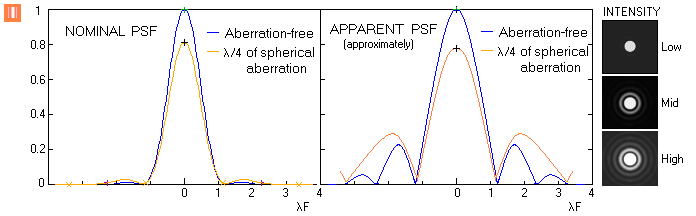The above refers to diffraction at a circular aperture. Aperture forms other than circular will produce different diffraction patterns. Two examples are square and triangular aperture, with their PSF, MTF and diffraction patterns shown below in comparison with the limiting circular aperture.

INSET J: With the intensity distribution of rectangular aperture for central intensity normalized to 1 given by I(r)=(sinc2α)(sinc2β), with sincα=sinα/α, α=πax/f and β=πby/f, for x and y the Cartesian coordinates in the image plane with the origin (zero) at the diffraction peak, a and b the lengths of the two sides of a rectangle, and f the focal length, normalized intensity distribution for square aperture is obtained by setting a=b. First minima, along either x or y axis, is at λf/a, with every next minima falling at this exact separation (the minimas follow straight lines running parallel with one or the other axis). The sinc value is undefined for zero angle, but since it approaches 1 as the angle approaches 0, 1 is its limit value.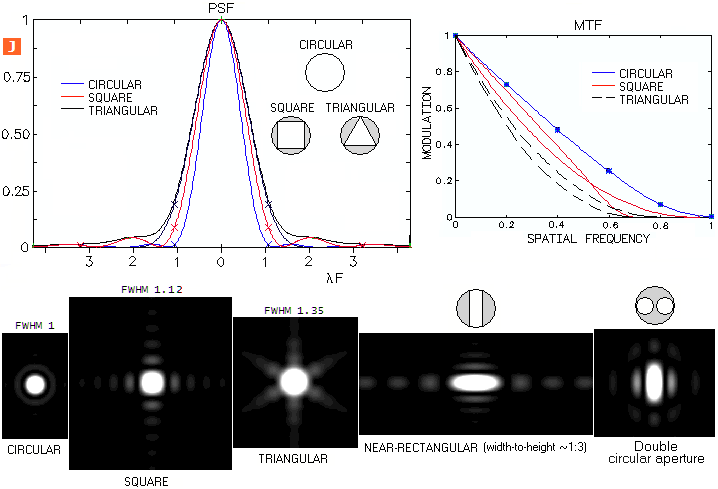Expectedly, central maxima of either square of triangular aperture fit is larger than for the limiting circular aperture. This results in less efficient contrast transfer and somewhat inferior limiting resolution (the double lines on the PSF and MTF plots are for the vertical/horizontal and 45° orientation for the square aperture, and for the vertical and horizontal orientation for triangular aperture). First minima of the square aperture is at
λF radius, with F defined by f/S, S being the aperture side; each successive minima is at the same λF separation from the previous one. More detailed structure of its central core would show as the square base of the central maxima gradually transforms into a round cross section toward the point of peak intensity, with the cross section at half maximum (FWHM) being nearly spherical. Central maxima of triangular aperture is only slightly hexagonal. Both, square and triangular aperture have multiple, radially distributed spike-like successive maximas, result of the "missing" aperture portions relative to the full circular one (shaded areas within the circles inside the PSF plot). These portions act like inverse apertures, effectively superimposing their diffraction pattern over that of the full circular aperture as an inverse energy contribution. Since these apertures are elongated, their diffraction patterns are extended in the direction perpendicular to the aperture extension, forming the spike-like intensity distribution around central maxima (diffraction pattern of an elongated rectangular aperture that would result from from rectangular aperture fit of about 3-to-1 height-to-width ratio is shown). The last pattern is produced by a double circular aperture. Since the number of segments over the central disc is given by N=2.44s/D, s being the center separation of the two apertures, the pattern shows only the beginning of segmentation (s~0.5D).

(1)Actual astronomical point-sources - stars - are spatially extended, with the wave emission phase varying from one point to another, and also in time. Thus, strictly talking, they emit incoherent light. However, according to Van Cittert-Zernike Theorem - stating that the degree of spatial coherence of near-monochromatic light equals the normalized (to 1) PSF of the source - the degree of coherence between two wave trains from a star will diminish from 1 to 0 as their angular separation in the image plane increases from zero to 1.22λ/S, where S is their separation in the aperture. In other words, the complex degree of coherence  ɤ for a star of angular size A in radians is 1 for two points in the aperture nearly coinciding, diminishing to zero as their linear separation increases to 1.22λ/A. Hence even the largest star angularly, Betelgeuse (slightly less than0.045 arc seconds in diameter @550nm), would have its ɤ value change only from 1 to over 0.99 for two rays from the opposite ends of S=D=200mm aperture (1.38 arc seconds Airy disc diameter). On the other hand, Jupiter with its average 40 arc seconds angular diameter would have ɤ falling from 1 to 0 already at nearly 1/60 of its radius. Since boundary waves become incoherent when angular radius of the object equals that of the Airy disc - assuming, for simplicity, circular object - the corresponding aperture (so called "coherence radius") is given by Dc=1.22λ/A for the object's angular diameter A in radians, or Dc=251,643λ/A, for A in arc seconds, with Dc being in the same units as λ (when two slits are placed at separation equaling D, the fringes in their interference pattern disappear, which is how Michelson had determined first angular diameter of a star - Betelgeuse, from Dc=3070mm for λ=0.00057mm). At this point, the average coherence, as the averaged ɤ value, equals intensity averaged over the Airy disc (as flux divided by area, normalized to the central intensity), which is 0.23 for perfect aperture. Hence, it is a low-coherence, partly incoherent light. Note that Van Cittert-Zernike Theorem strictly applies to near-monochromatic light, so the fact that stars are polychromatic sources also needs to be taken into account. As Inset H shows, widening of the spectral range lowers light coherency, until it becomes incoherent as the range approaches the mean wavelength.
(2) Value in the brackets is the normalized wave field, i.e.  sum of the individual wave amplitudes; since wave energy, given by the Poynting vector (vector product of the magnetic and electric field vectors), is proportional to the product of the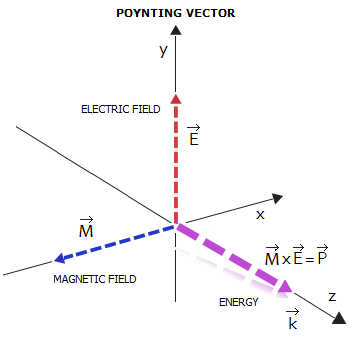electric and magnetic field component - i.e. to a product of the respective electric and magnetic field amplitudes - with the two being in a constant relation, it is also proportional to the amplitude squared. Incident wave energy is called irradiance. The Bessel function, which can be used to express the sum of phase (i.e. amplitude as sine/cosine function) contributions from the points on the wavefront in this form, replaces much more involved direct integration. Note that the PSF expression with Bessel function is not defined for r=0 (thus t=0), but it approaches 1 as r and t approach zero.